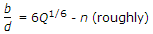# Civil Engineering - Hydraulics

### Exercise :: Hydraulics - Section 3

21.

Uniform flow is said to occur when

 A. size and shape of the cross-section in a particular length remain constant B. size and shape of the cross-section change along a length C. frictional loss in the particular length of the channel will the more than the drop in its elevation D. frictional loss in the particular length of the channel, will be less than the drop in elevation.

Explanation:

No answer description available for this question. Let us discuss.

22.

For the most economical trapezoidal section of a channel with regards to discharge, the required condition, is

 A. half of top width = sloping side B. hydraulic depth = half the depth C.D. perpendiculars drawn from the centre of the top width on to the bottom sloping side, are all equal E. all the above.

Explanation:

No answer description available for this question. Let us discuss.

23.

Molecules of fluids get attracted due to

 A. capillarity action B. surface tension C. adhesion D. cohesion E. none of these.

Explanation:

No answer description available for this question. Let us discuss.

24.

Cappoletti weir is a

 A. rectangular weir whose length is kept 3 times the height of the water above sill B. triangular weir whose notch angle is 90° C. trapezoidal weir, whose sides slope 1 horizontal to 2 verticals D. a combination of rectangular and triangular weirs.

Explanation:

No answer description available for this question. Let us discuss.

25.

On an inclined plane, centre of pressure is located

 A. at the centroid B. above the centroid C. below the centroid D. anywhere.